Скачать презентацию Nuclear Chemistry selected topics Introduction and Important

c91a345b30e75c305cf7f30bd0e8eb13.ppt

• Количество слайдов: 64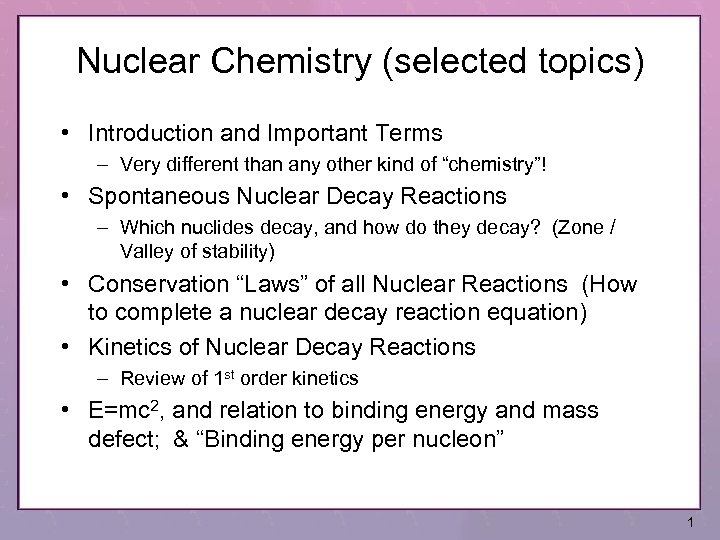Nuclear Chemistry (selected topics) • Introduction and Important Terms – Very different than any other kind of “chemistry”! • Spontaneous Nuclear Decay Reactions – Which nuclides decay, and how do they decay? (Zone / Valley of stability) • Conservation “Laws” of all Nuclear Reactions (How to complete a nuclear decay reaction equation) • Kinetics of Nuclear Decay Reactions – Review of 1 st order kinetics • E=mc 2, and relation to binding energy and mass defect; & “Binding energy per nucleon” 1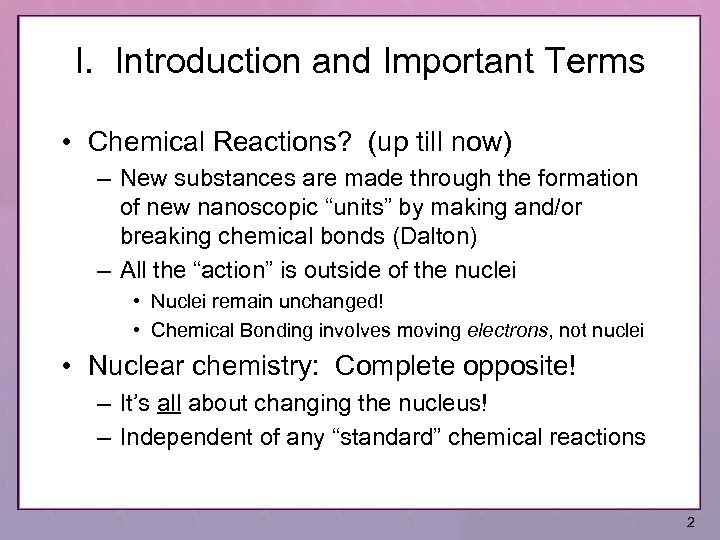I. Introduction and Important Terms • Chemical Reactions? (up till now) – New substances are made through the formation of new nanoscopic “units” by making and/or breaking chemical bonds (Dalton) – All the “action” is outside of the nuclei • Nuclei remain unchanged! • Chemical Bonding involves moving electrons, not nuclei • Nuclear chemistry: Complete opposite! – It’s all about changing the nucleus! – Independent of any “standard” chemical reactions 2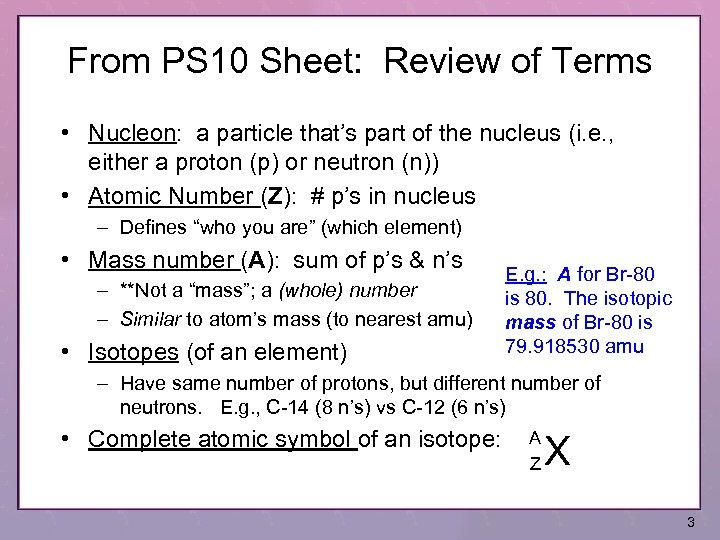From PS 10 Sheet: Review of Terms • Nucleon: a particle that’s part of the nucleus (i. e. , either a proton (p) or neutron (n)) • Atomic Number (Z): # p’s in nucleus – Defines “who you are” (which element) • Mass number (A): sum of p’s & n’s – **Not a “mass”; a (whole) number – Similar to atom’s mass (to nearest amu) • Isotopes (of an element) E. g. : A for Br-80 is 80. The isotopic mass of Br-80 is 79. 918530 amu – Have same number of protons, but different number of neutrons. E. g. , C-14 (8 n’s) vs C-12 (6 n’s) • Complete atomic symbol of an isotope: A Z X 3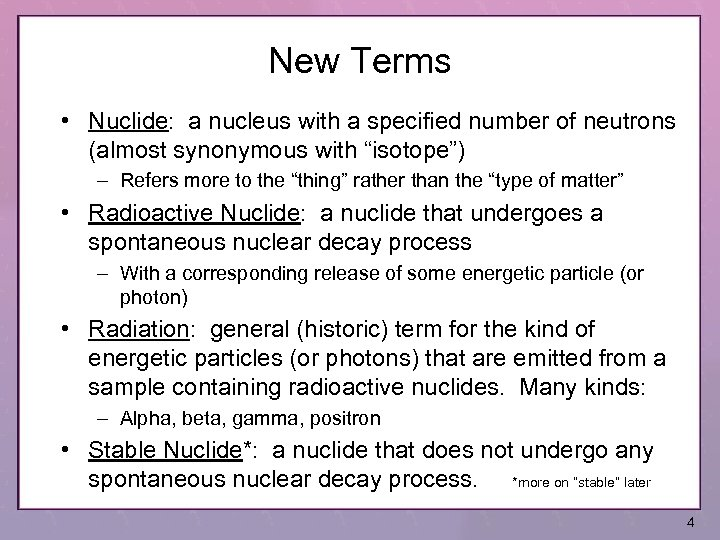New Terms • Nuclide: a nucleus with a specified number of neutrons (almost synonymous with “isotope”) – Refers more to the “thing” rather than the “type of matter” • Radioactive Nuclide: a nuclide that undergoes a spontaneous nuclear decay process – With a corresponding release of some energetic particle (or photon) • Radiation: general (historic) term for the kind of energetic particles (or photons) that are emitted from a sample containing radioactive nuclides. Many kinds: – Alpha, beta, gamma, positron • Stable Nuclide*: a nuclide that does not undergo any spontaneous nuclear decay process. *more on “stable” later 4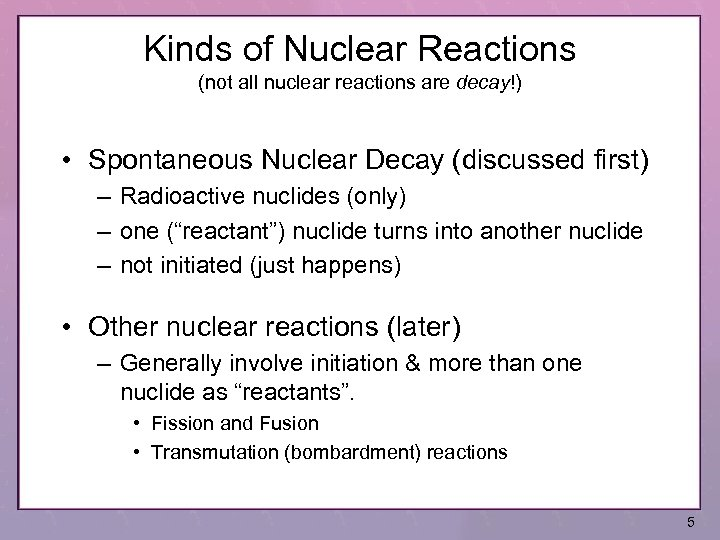Kinds of Nuclear Reactions (not all nuclear reactions are decay!) • Spontaneous Nuclear Decay (discussed first) – Radioactive nuclides (only) – one (“reactant”) nuclide turns into another nuclide – not initiated (just happens) • Other nuclear reactions (later) – Generally involve initiation & more than one nuclide as “reactants”. • Fission and Fusion • Transmutation (bombardment) reactions 5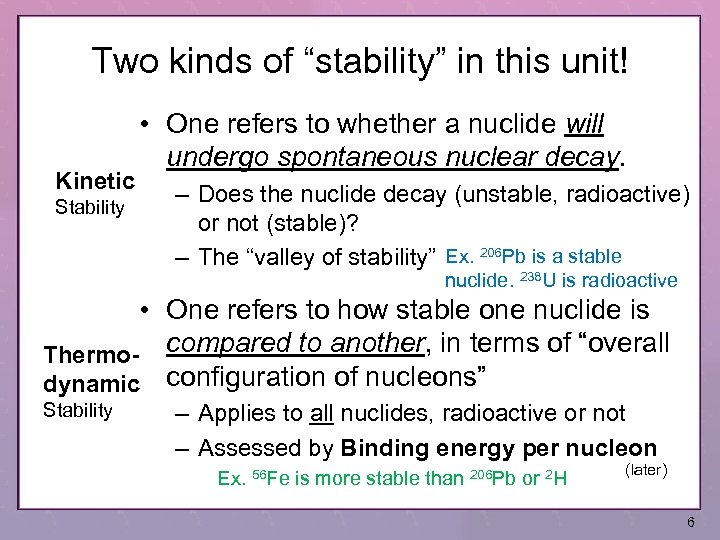Two kinds of “stability” in this unit! Kinetic Stability • One refers to whether a nuclide will undergo spontaneous nuclear decay. – Does the nuclide decay (unstable, radioactive) or not (stable)? – The “valley of stability” Ex. 206 Pb is a stable nuclide. 238 U is radioactive • One refers to how stable one nuclide is Thermo- compared to another, in terms of “overall dynamic configuration of nucleons” Stability – Applies to all nuclides, radioactive or not – Assessed by Binding energy per nucleon Ex. 56 Fe is more stable than 206 Pb or 2 H (later) 6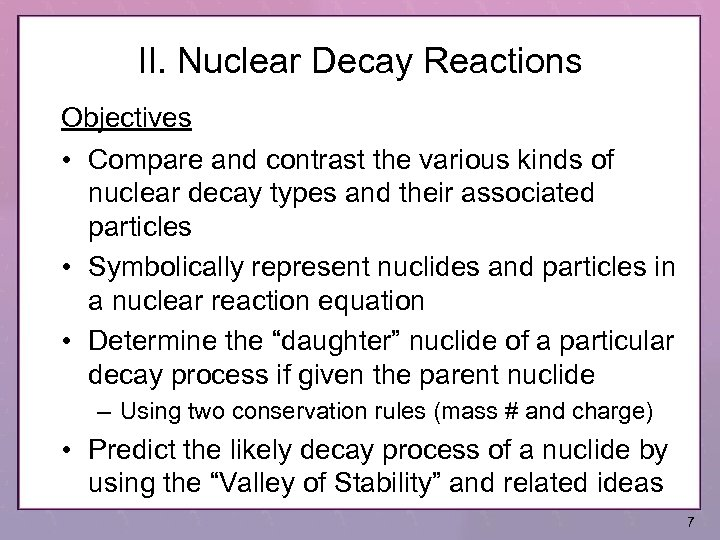II. Nuclear Decay Reactions Objectives • Compare and contrast the various kinds of nuclear decay types and their associated particles • Symbolically represent nuclides and particles in a nuclear reaction equation • Determine the “daughter” nuclide of a particular decay process if given the parent nuclide – Using two conservation rules (mass # and charge) • Predict the likely decay process of a nuclide by using the “Valley of Stability” and related ideas 7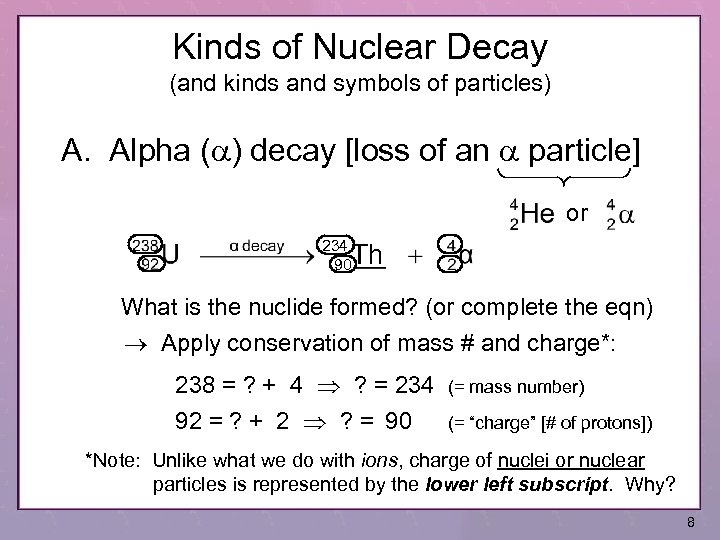Kinds of Nuclear Decay (and kinds and symbols of particles) A. Alpha (a) decay [loss of an a particle] or 234 90 Th What is the nuclide formed? (or complete the eqn) Apply conservation of mass # and charge*: 238 = ? + 4 ? = 234 92 = ? + 2 ? = 90 (= mass number) (= “charge” [# of protons]) *Note: Unlike what we do with ions, charge of nuclei or nuclear particles is represented by the lower left subscript. Why? 8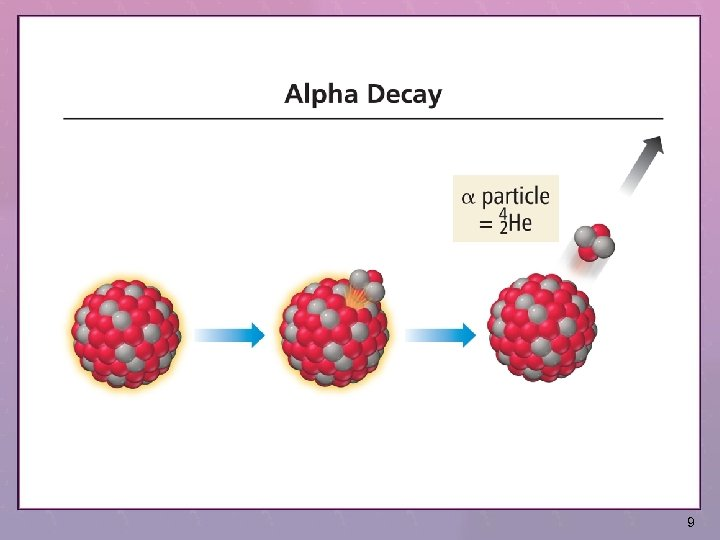9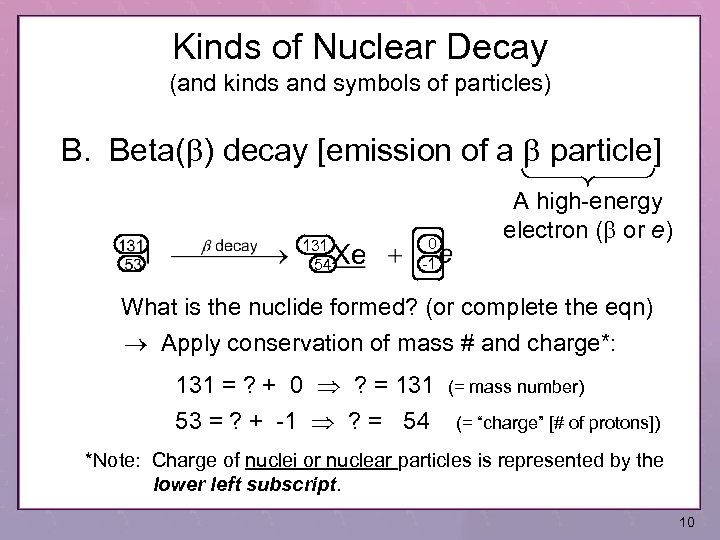Kinds of Nuclear Decay (and kinds and symbols of particles) B. Beta(b) decay [emission of a b particle] 131 54 Xe 0 -1 A high-energy electron (b or e) What is the nuclide formed? (or complete the eqn) Apply conservation of mass # and charge*: 131 = ? + 0 ? = 131 53 = ? + -1 ? = 54 (= mass number) (= “charge” [# of protons]) *Note: Charge of nuclei or nuclear particles is represented by the lower left subscript. 10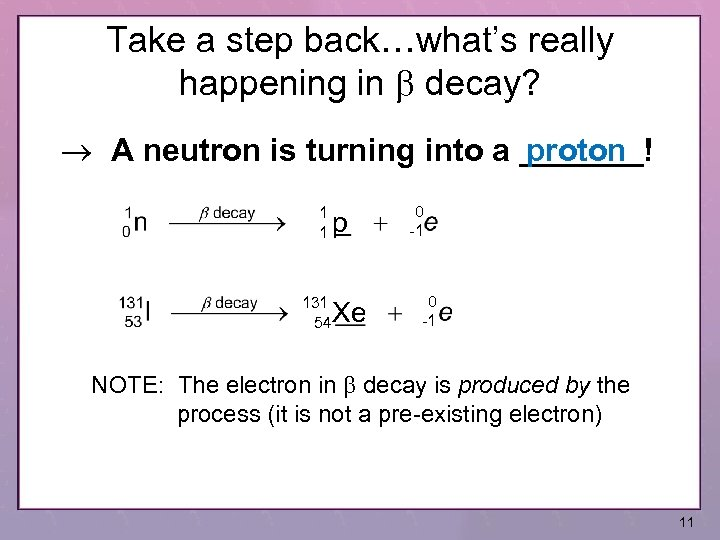Take a step back…what’s really happening in b decay? A neutron is turning into a _______! proton 1 1 131 54 p Xe 0 -1 NOTE: The electron in b decay is produced by the process (it is not a pre-existing electron) 11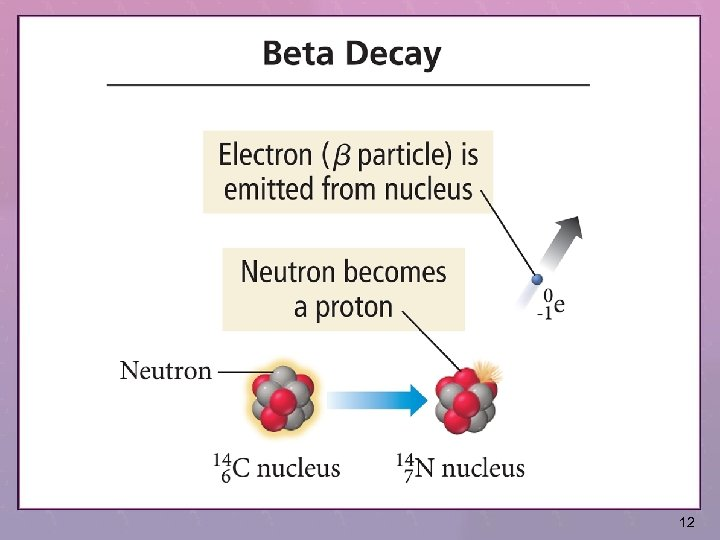12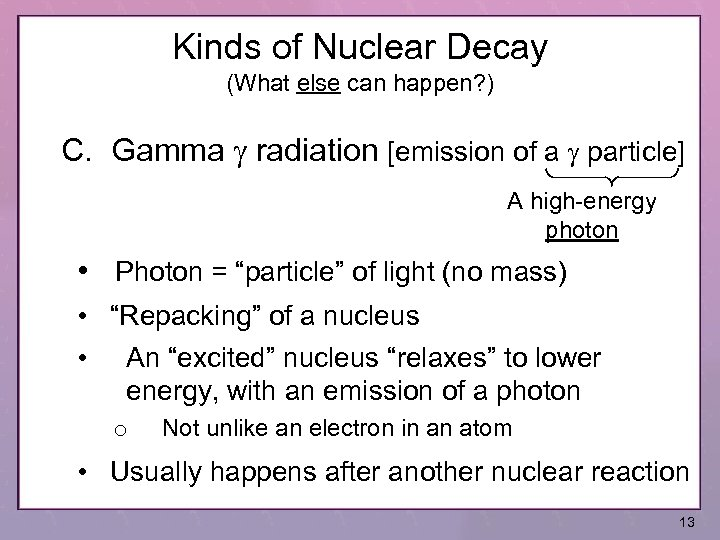Kinds of Nuclear Decay (What else can happen? ) C. Gamma g radiation [emission of a g particle] A high-energy photon • Photon = “particle” of light (no mass) • “Repacking” of a nucleus • An “excited” nucleus “relaxes” to lower energy, with an emission of a photon o Not unlike an electron in an atom • Usually happens after another nuclear reaction 13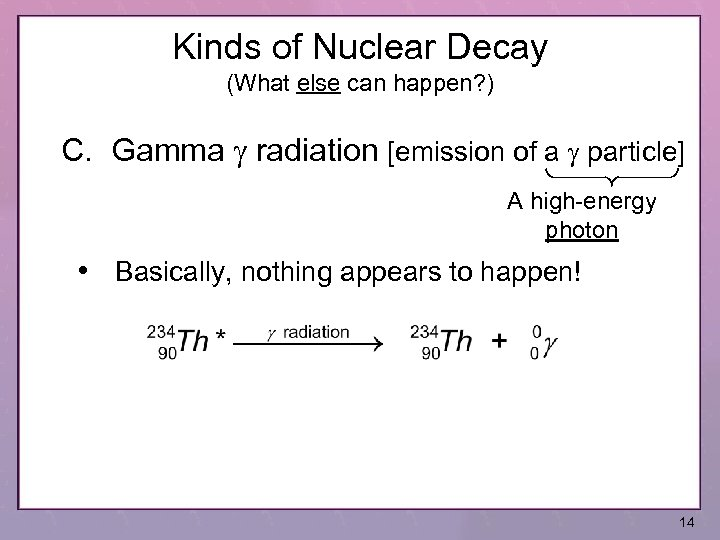Kinds of Nuclear Decay (What else can happen? ) C. Gamma g radiation [emission of a g particle] A high-energy photon • Basically, nothing appears to happen! 14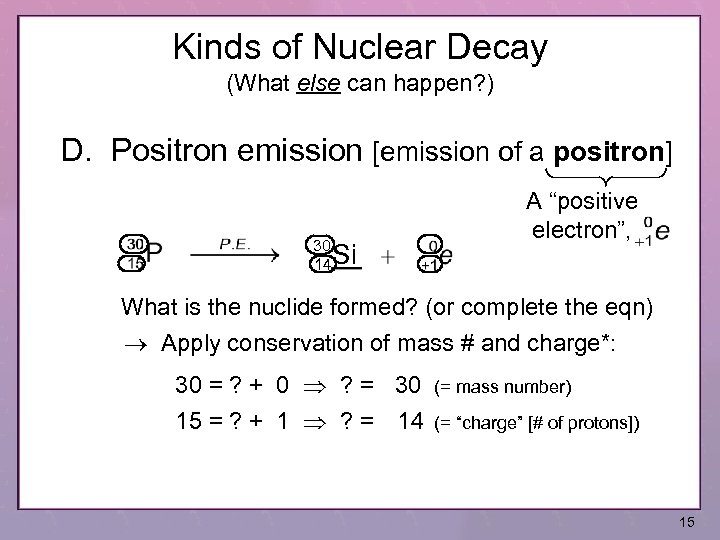Kinds of Nuclear Decay (What else can happen? ) D. Positron emission [emission of a positron] 30 14 Si A “positive electron”, What is the nuclide formed? (or complete the eqn) Apply conservation of mass # and charge*: 30 = ? + 0 ? = 30 15 = ? + 1 ? = 14 (= mass number) (= “charge” [# of protons]) 15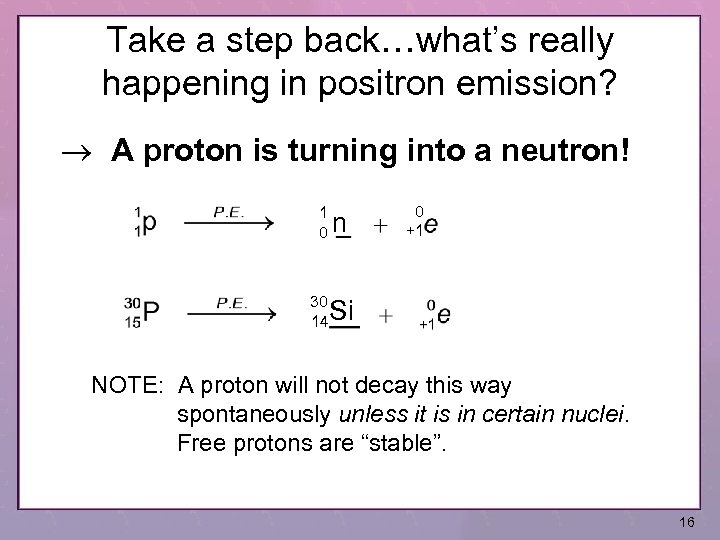Take a step back…what’s really happening in positron emission? A proton is turning into a neutron! 1 0 n 30 14 0 +1 Si NOTE: A proton will not decay this way spontaneously unless it is in certain nuclei. Free protons are “stable”. 16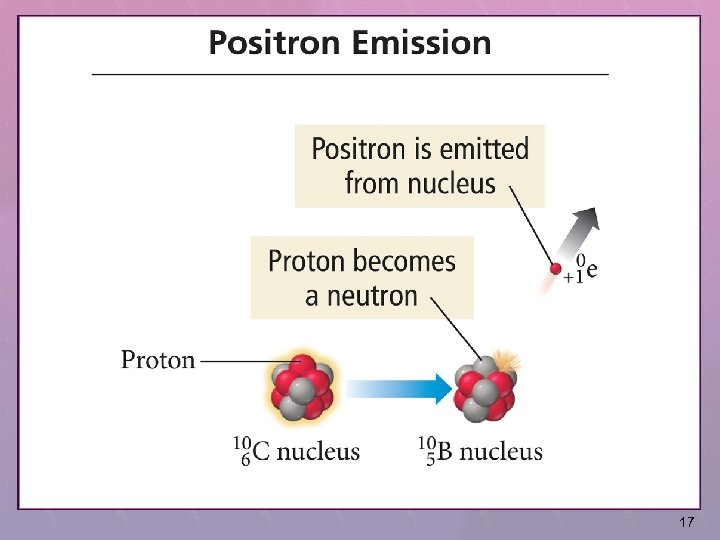17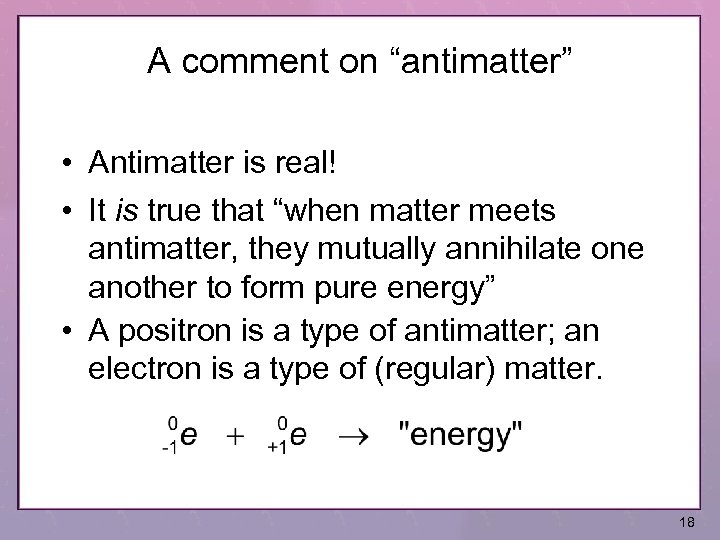A comment on “antimatter” • Antimatter is real! • It is true that “when matter meets antimatter, they mutually annihilate one another to form pure energy” • A positron is a type of antimatter; an electron is a type of (regular) matter. 18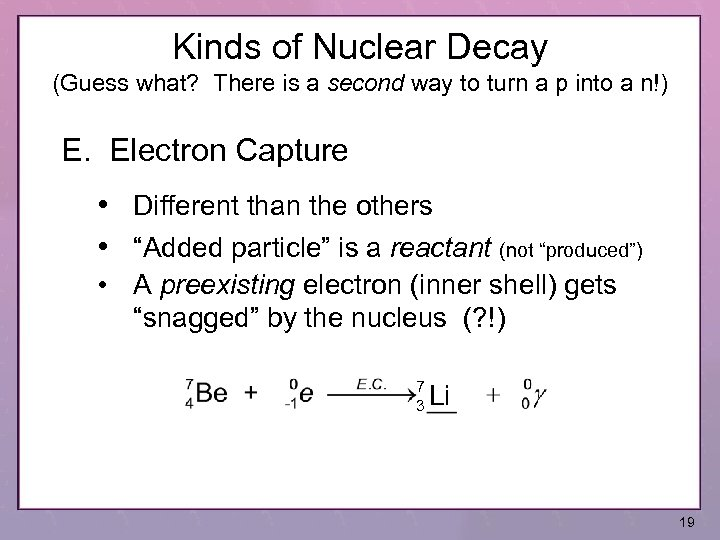Kinds of Nuclear Decay (Guess what? There is a second way to turn a p into a n!) E. Electron Capture • Different than the others • “Added particle” is a reactant (not “produced”) • A preexisting electron (inner shell) gets “snagged” by the nucleus (? !) 7 3 Li 19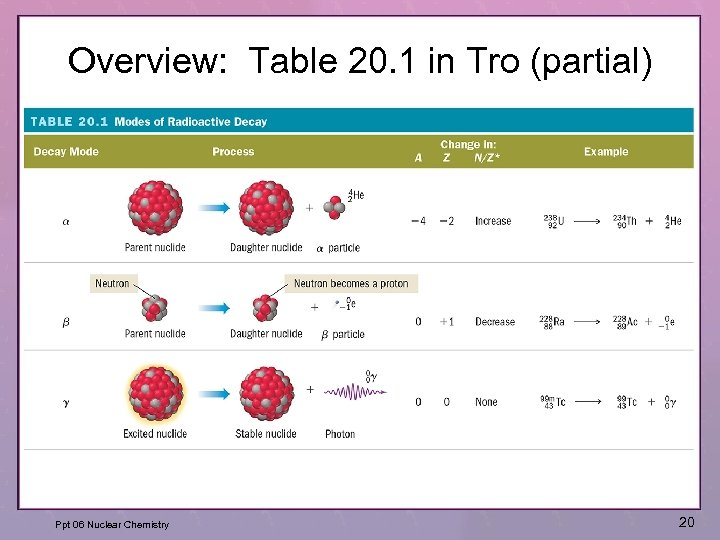Overview: Table 20. 1 in Tro (partial) Ppt 06 Nuclear Chemistry 20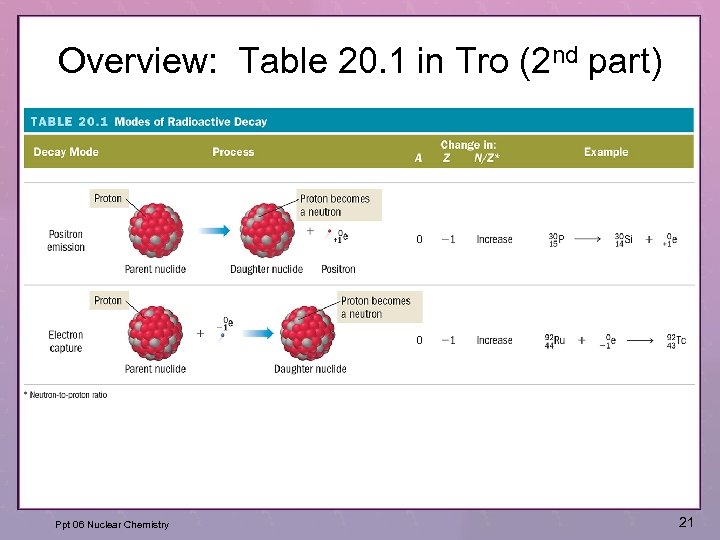Overview: Table 20. 1 in Tro (2 nd part) Ppt 06 Nuclear Chemistry 21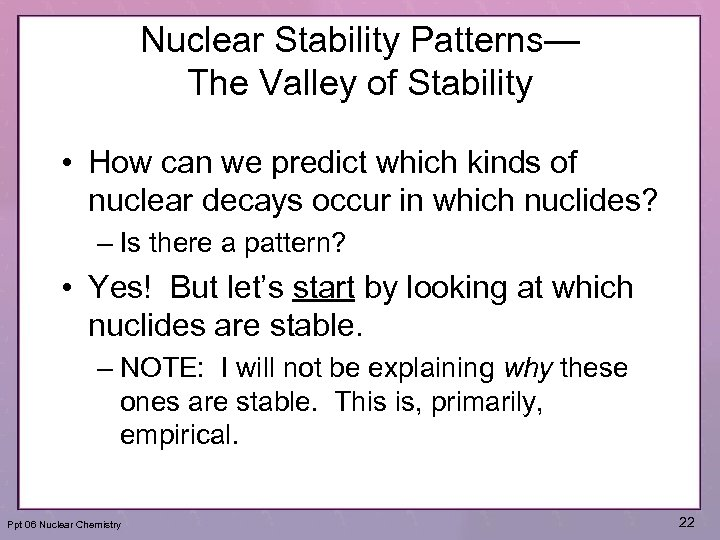Nuclear Stability Patterns— The Valley of Stability • How can we predict which kinds of nuclear decays occur in which nuclides? – Is there a pattern? • Yes! But let’s start by looking at which nuclides are stable. – NOTE: I will not be explaining why these ones are stable. This is, primarily, empirical. Ppt 06 Nuclear Chemistry 22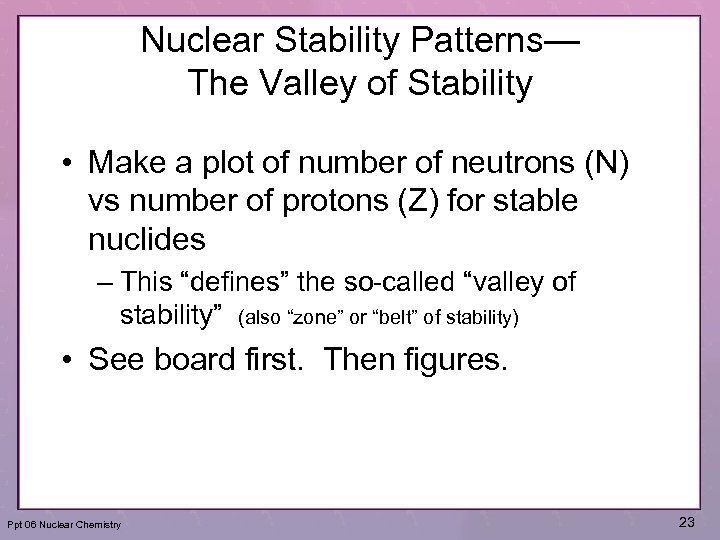Nuclear Stability Patterns— The Valley of Stability • Make a plot of number of neutrons (N) vs number of protons (Z) for stable nuclides – This “defines” the so-called “valley of stability” (also “zone” or “belt” of stability) • See board first. Then figures. Ppt 06 Nuclear Chemistry 23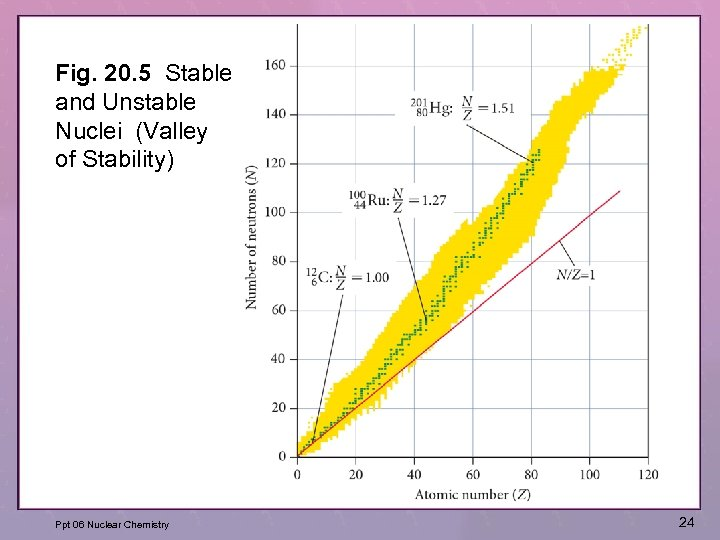Fig. 20. 5 Stable and Unstable Nuclei (Valley of Stability) Ppt 06 Nuclear Chemistry 24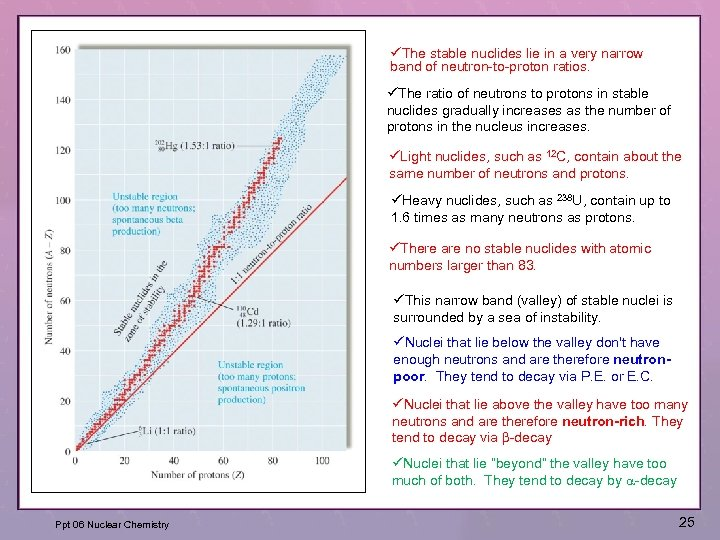üThe stable nuclides lie in a very narrow band of neutron-to-proton ratios. üThe ratio of neutrons to protons in stable nuclides gradually increases as the number of protons in the nucleus increases. üLight nuclides, such as 12 C, contain about the same number of neutrons and protons. üHeavy nuclides, such as 238 U, contain up to 1. 6 times as many neutrons as protons. üThere are no stable nuclides with atomic numbers larger than 83. üThis narrow band (valley) of stable nuclei is surrounded by a sea of instability. üNuclei that lie below the valley don't have enough neutrons and are therefore neutronpoor. They tend to decay via P. E. or E. C. üNuclei that lie above the valley have too many neutrons and are therefore neutron-rich. They tend to decay via b-decay üNuclei that lie “beyond” the valley have too much of both. They tend to decay by a-decay Ppt 06 Nuclear Chemistry 25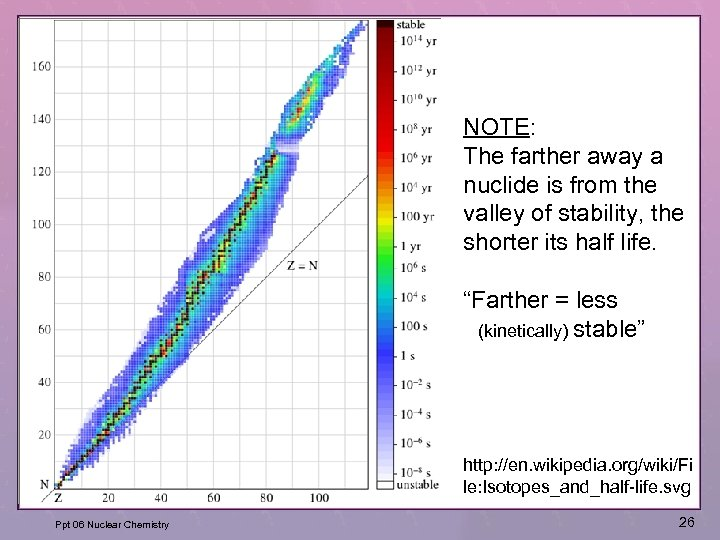NOTE: The farther away a nuclide is from the valley of stability, the shorter its half life. “Farther = less (kinetically) stable” http: //en. wikipedia. org/wiki/Fi le: Isotopes_and_half-life. svg Ppt 06 Nuclear Chemistry 26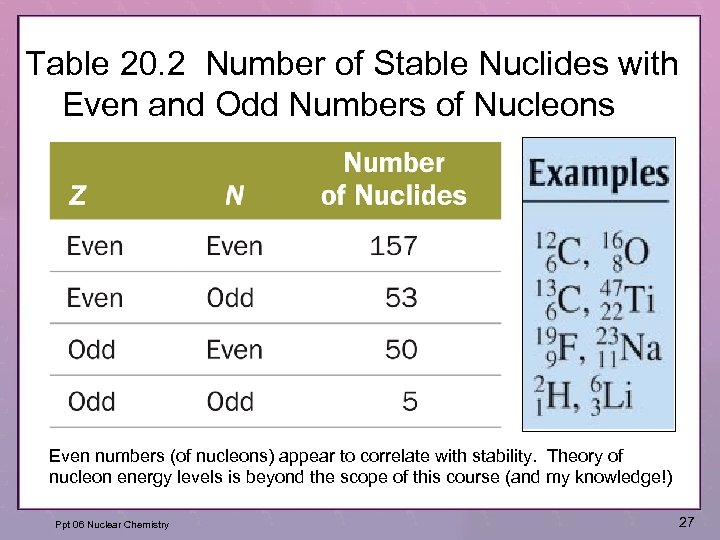Table 20. 2 Number of Stable Nuclides with Even and Odd Numbers of Nucleons Even numbers (of nucleons) appear to correlate with stability. Theory of nucleon energy levels is beyond the scope of this course (and my knowledge!) Ppt 06 Nuclear Chemistry 27More from the web… • http: //en. wikipedia. org/wiki/Isotope • http: //atom. kaeri. re. kr/ 28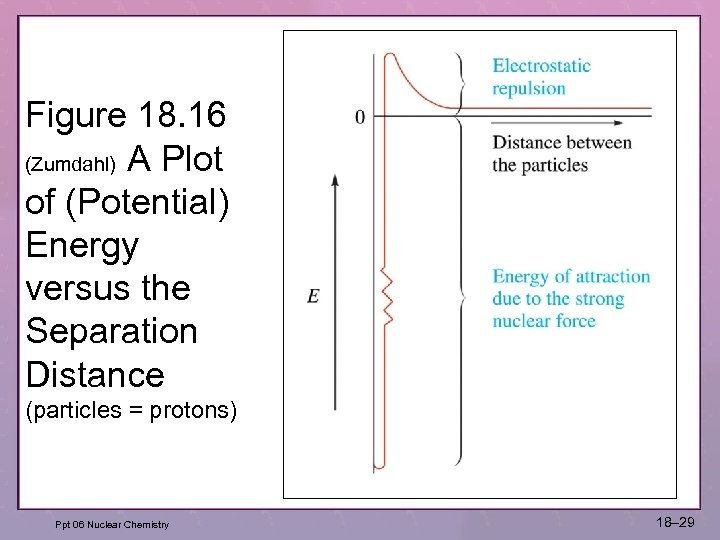Figure 18. 16 (Zumdahl) A Plot of (Potential) Energy versus the Separation Distance (particles = protons) Ppt 06 Nuclear Chemistry 18– 29 29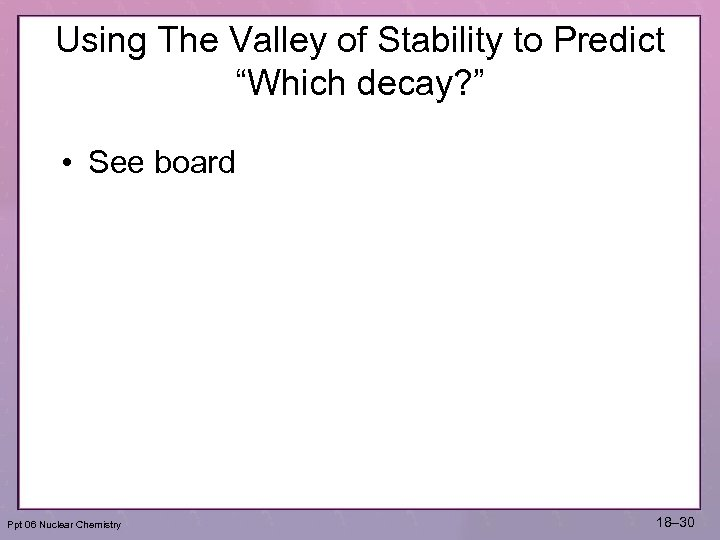Using The Valley of Stability to Predict “Which decay? ” • See board Ppt 06 Nuclear Chemistry 18– 30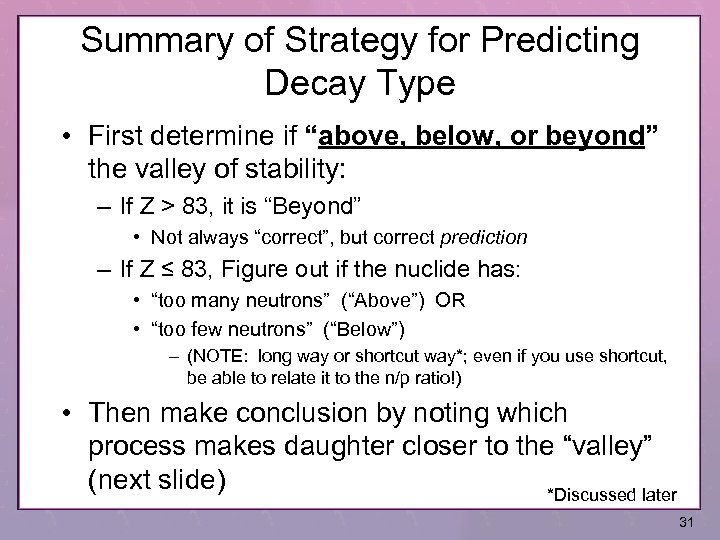Summary of Strategy for Predicting Decay Type • First determine if “above, below, or beyond” the valley of stability: – If Z > 83, it is “Beyond” • Not always “correct”, but correct prediction – If Z ≤ 83, Figure out if the nuclide has: • “too many neutrons” (“Above”) OR • “too few neutrons” (“Below”) – (NOTE: long way or shortcut way*; even if you use shortcut, be able to relate it to the n/p ratio!) • Then make conclusion by noting which process makes daughter closer to the “valley” (next slide) *Discussed later 31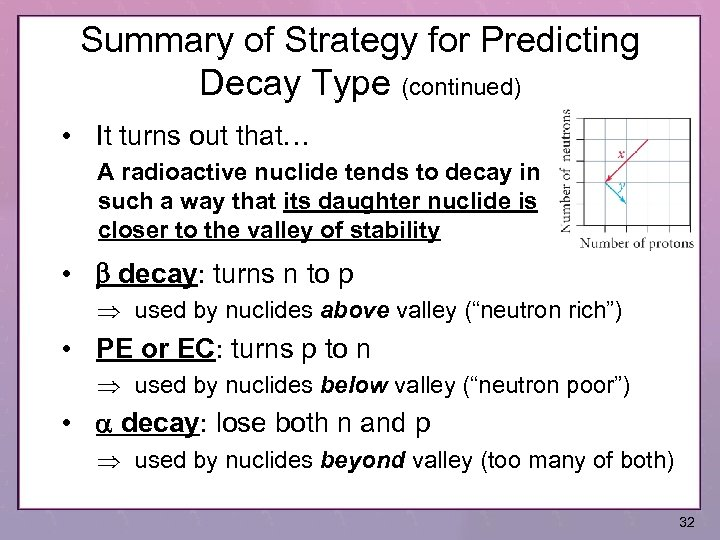Summary of Strategy for Predicting Decay Type (continued) • It turns out that… A radioactive nuclide tends to decay in such a way that its daughter nuclide is closer to the valley of stability • b decay: turns n to p used by nuclides above valley (“neutron rich”) • PE or EC: turns p to n used by nuclides below valley (“neutron poor”) • a decay: lose both n and p used by nuclides beyond valley (too many of both) 32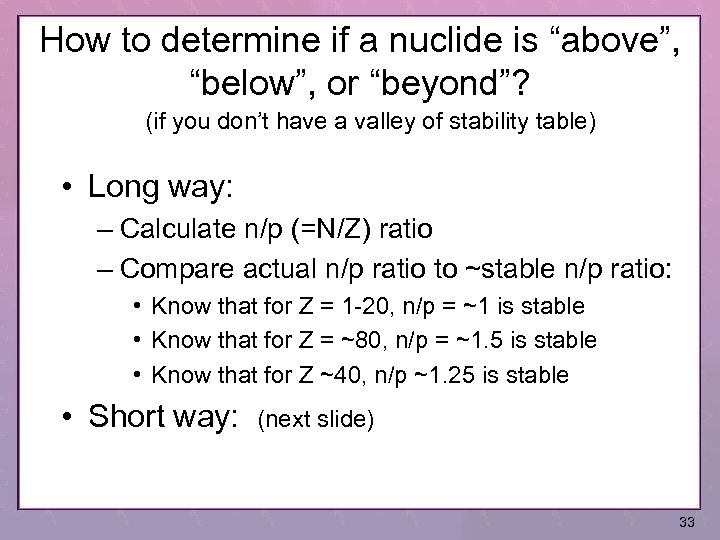How to determine if a nuclide is “above”, “below”, or “beyond”? (if you don’t have a valley of stability table) • Long way: – Calculate n/p (=N/Z) ratio – Compare actual n/p ratio to ~stable n/p ratio: • Know that for Z = 1 -20, n/p = ~1 is stable • Know that for Z = ~80, n/p = ~1. 5 is stable • Know that for Z ~40, n/p ~1. 25 is stable • Short way: (next slide) 33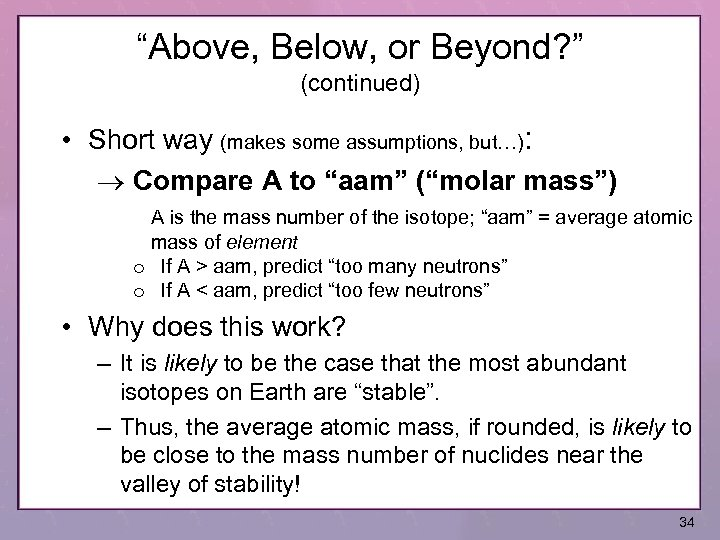“Above, Below, or Beyond? ” (continued) • Short way (makes some assumptions, but…): Compare A to “aam” (“molar mass”) A is the mass number of the isotope; “aam” = average atomic mass of element o If A > aam, predict “too many neutrons” o If A < aam, predict “too few neutrons” • Why does this work? – It is likely to be the case that the most abundant isotopes on Earth are “stable”. – Thus, the average atomic mass, if rounded, is likely to be close to the mass number of nuclides near the valley of stability! 34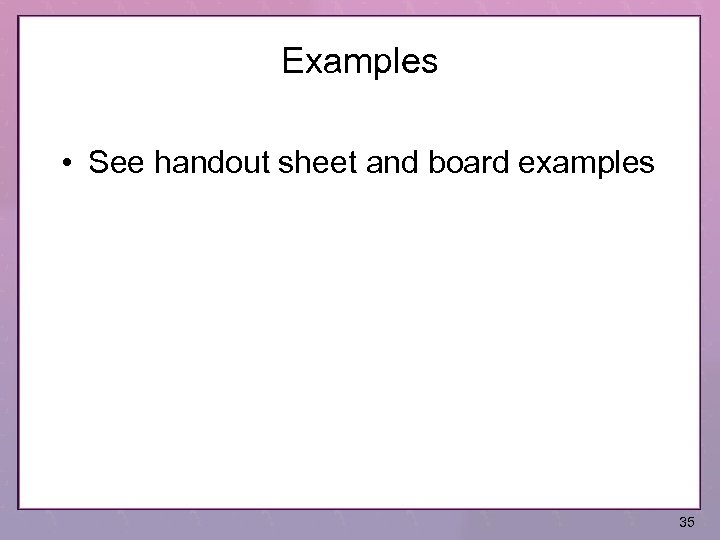Examples • See handout sheet and board examples 35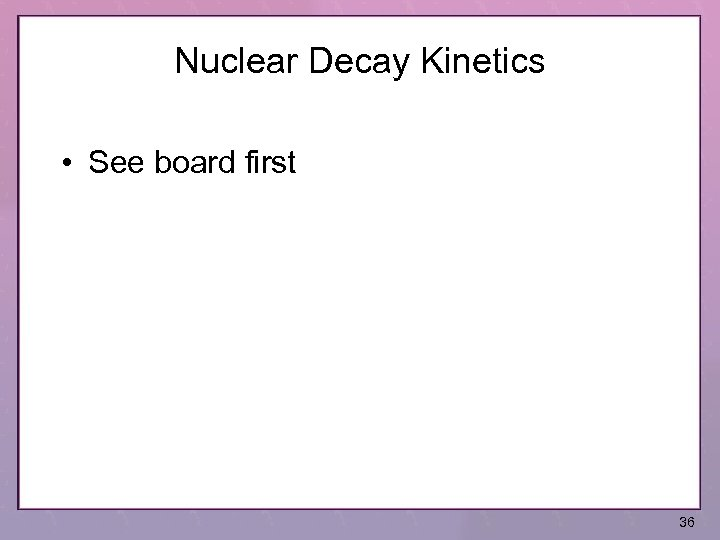Nuclear Decay Kinetics • See board first 36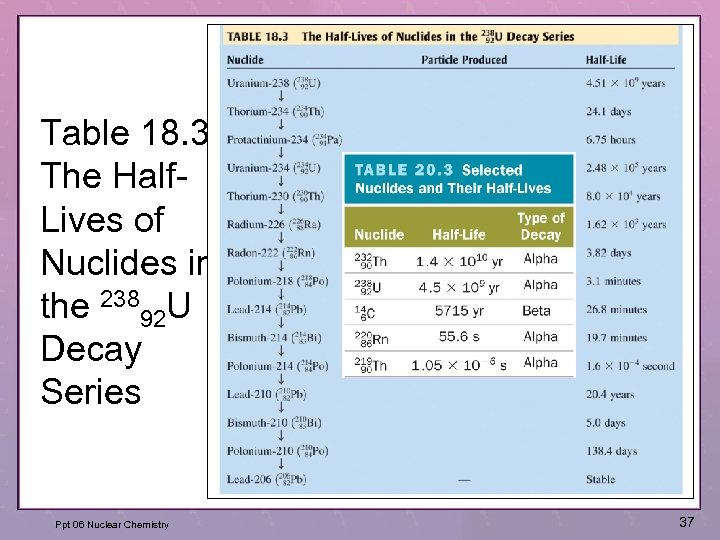Table 18. 3 The Half. Lives of Nuclides in the 23892 U Decay Series Ppt 06 Nuclear Chemistry 37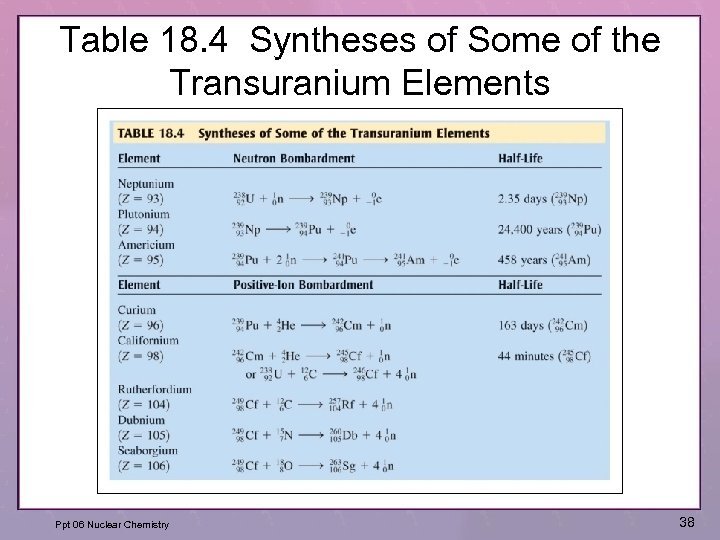Table 18. 4 Syntheses of Some of the Transuranium Elements Ppt 06 Nuclear Chemistry 38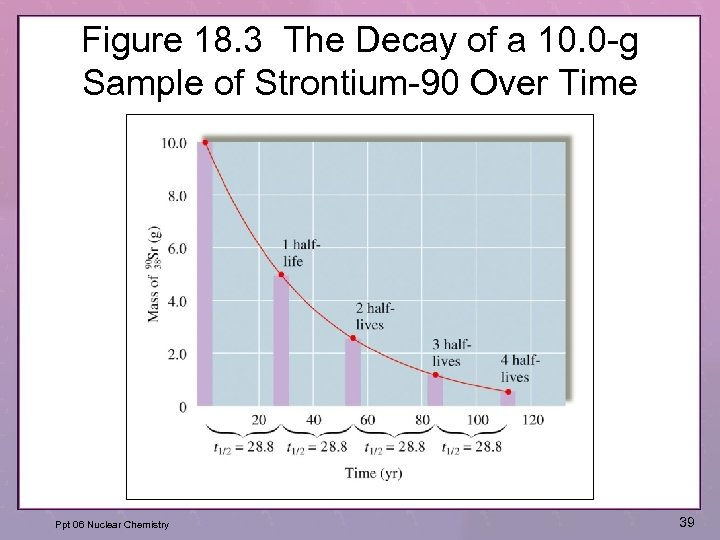Figure 18. 3 The Decay of a 10. 0 -g Sample of Strontium-90 Over Time Ppt 06 Nuclear Chemistry 39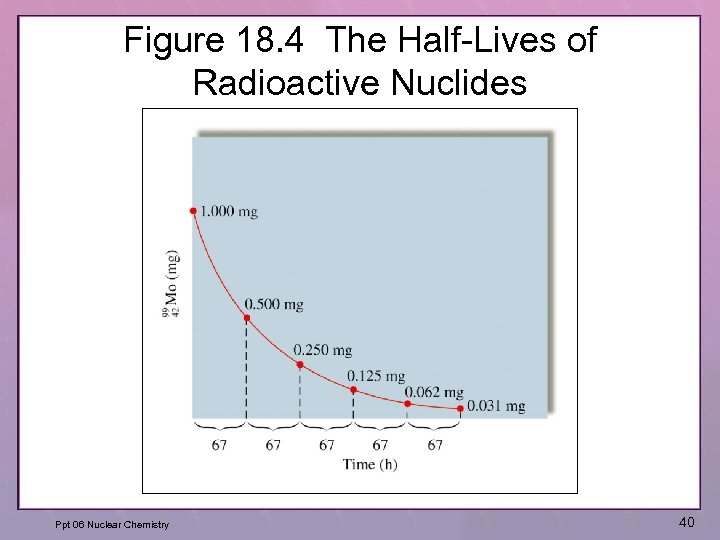Figure 18. 4 The Half-Lives of Radioactive Nuclides Ppt 06 Nuclear Chemistry 40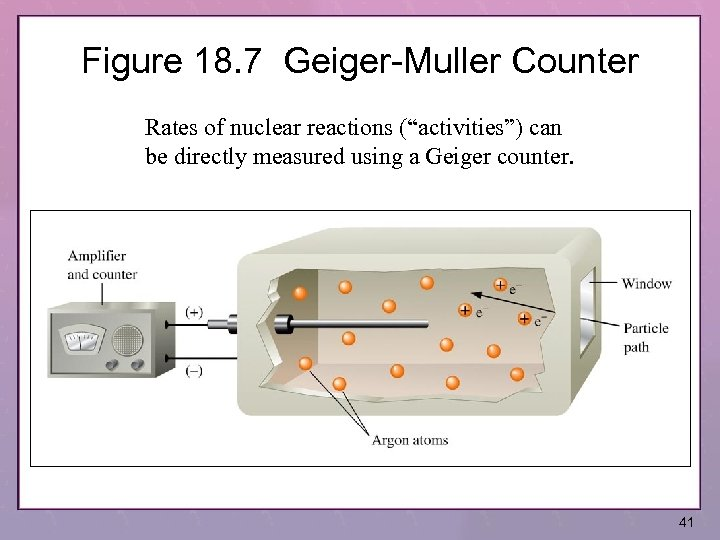Figure 18. 7 Geiger-Muller Counter Rates of nuclear reactions (“activities”) can be directly measured using a Geiger counter. 41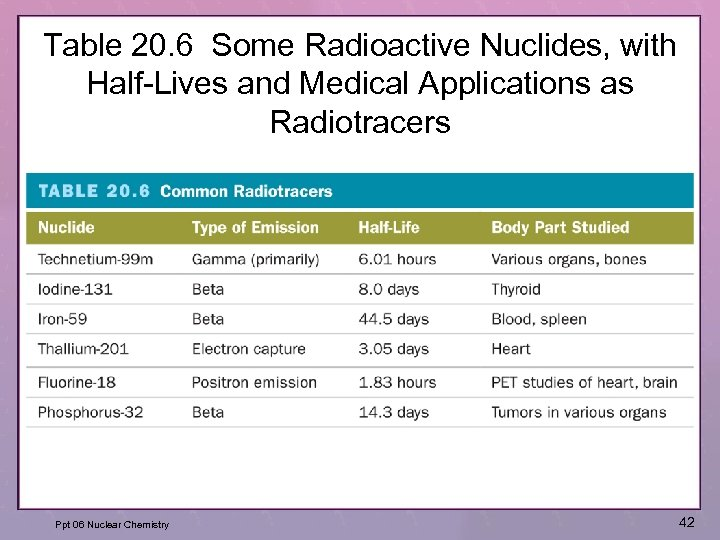Table 20. 6 Some Radioactive Nuclides, with Half-Lives and Medical Applications as Radiotracers Ppt 06 Nuclear Chemistry 42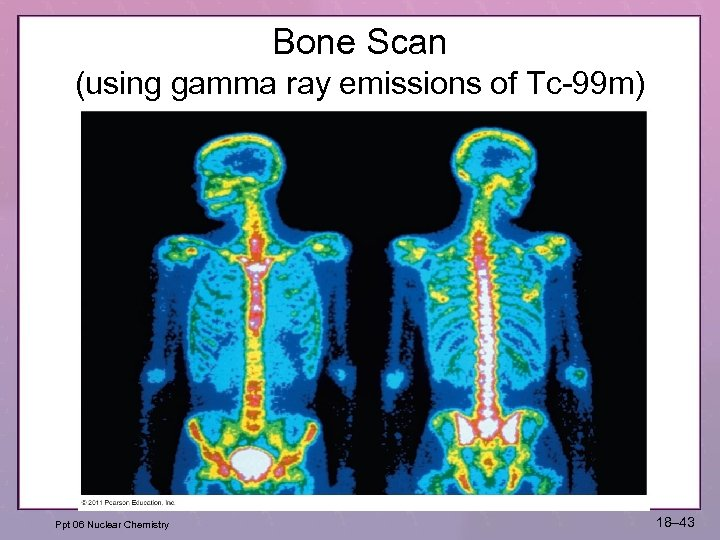Bone Scan (using gamma ray emissions of Tc-99 m) Ppt 06 Nuclear Chemistry 18– 43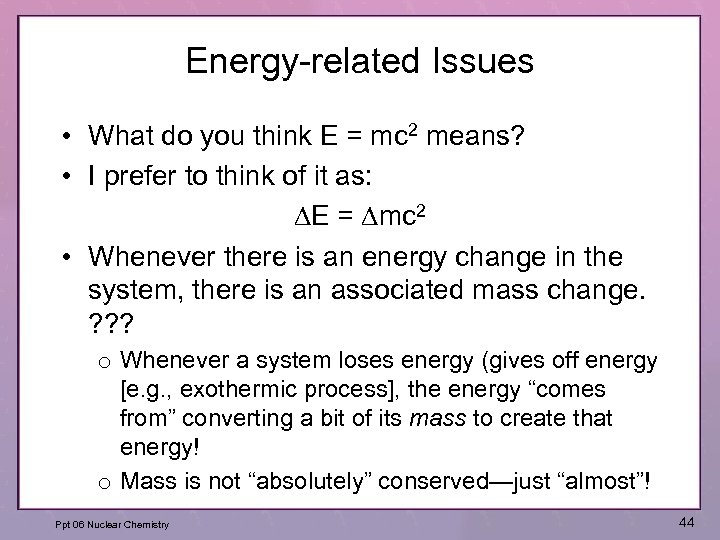Energy-related Issues • What do you think E = mc 2 means? • I prefer to think of it as: DE = Dmc 2 • Whenever there is an energy change in the system, there is an associated mass change. ? ? ? o Whenever a system loses energy (gives off energy [e. g. , exothermic process], the energy “comes from” converting a bit of its mass to create that energy! o Mass is not “absolutely” conserved—just “almost”! Ppt 06 Nuclear Chemistry 44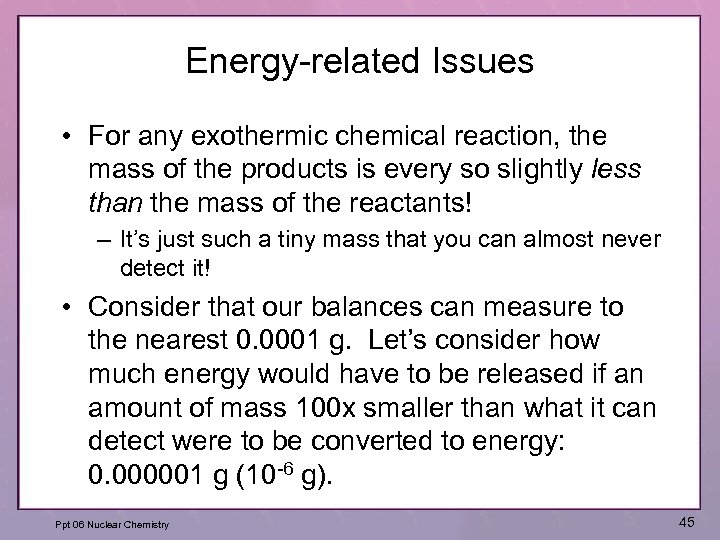Energy-related Issues • For any exothermic chemical reaction, the mass of the products is every so slightly less than the mass of the reactants! – It’s just such a tiny mass that you can almost never detect it! • Consider that our balances can measure to the nearest 0. 0001 g. Let’s consider how much energy would have to be released if an amount of mass 100 x smaller than what it can detect were to be converted to energy: 0. 000001 g (10 -6 g). Ppt 06 Nuclear Chemistry 45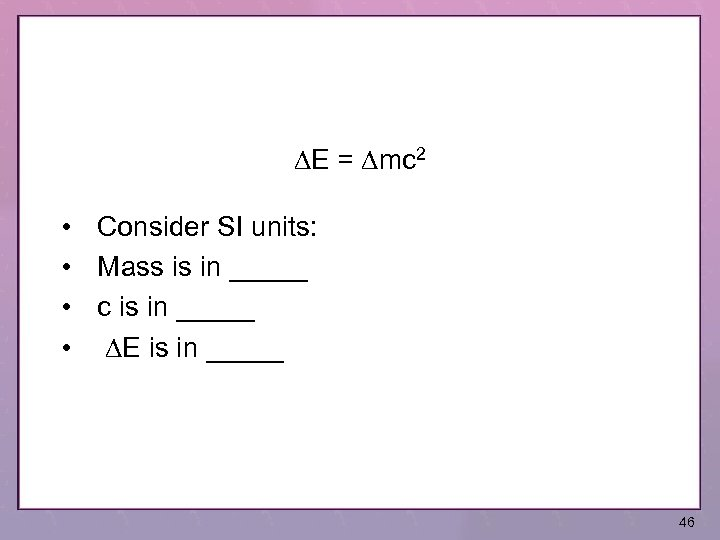DE = Dmc 2 • Consider SI units: • Mass is in _____ • c is in _____ • DE is in _____ 46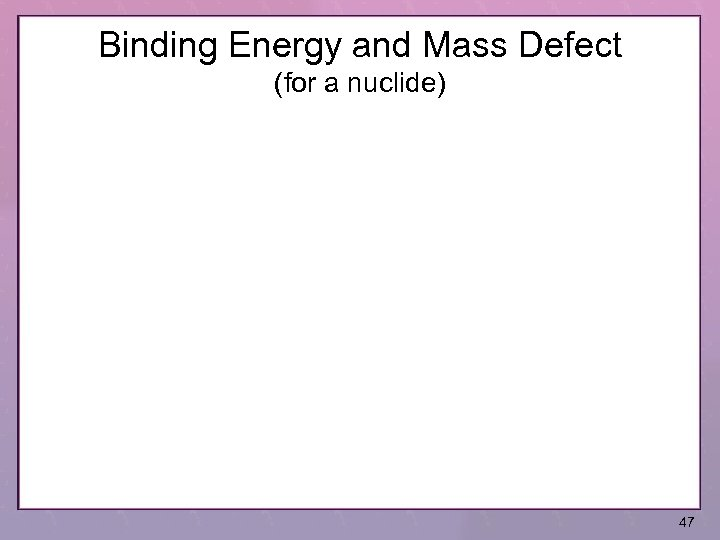Binding Energy and Mass Defect (for a nuclide) 47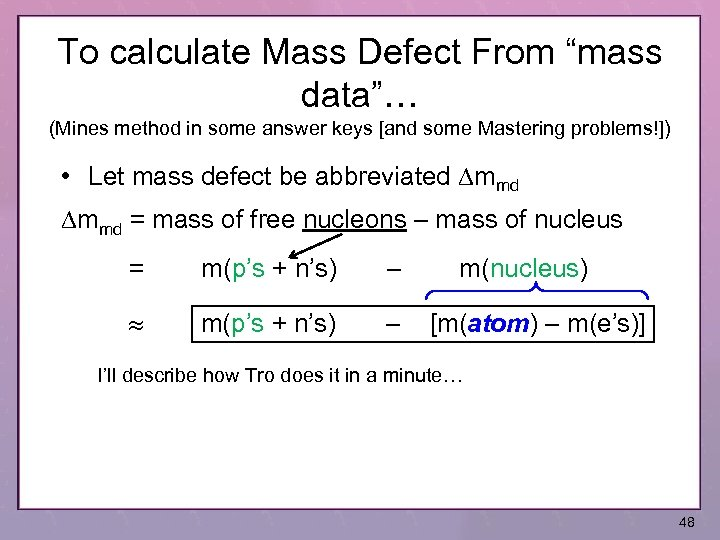To calculate Mass Defect From “mass data”… (Mines method in some answer keys [and some Mastering problems!]) • Let mass defect be abbreviated Dmmd = mass of free nucleons – mass of nucleus = m(p’s + n’s) – m(nucleus) [m(atom) – m(e’s)] I’ll describe how Tro does it in a minute… 48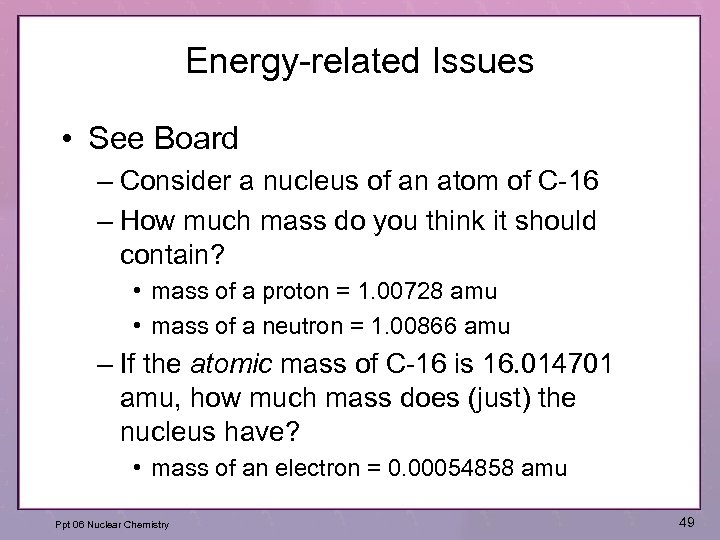Energy-related Issues • See Board – Consider a nucleus of an atom of C-16 – How much mass do you think it should contain? • mass of a proton = 1. 00728 amu • mass of a neutron = 1. 00866 amu – If the atomic mass of C-16 is 16. 014701 amu, how much mass does (just) the nucleus have? • mass of an electron = 0. 00054858 amu Ppt 06 Nuclear Chemistry 49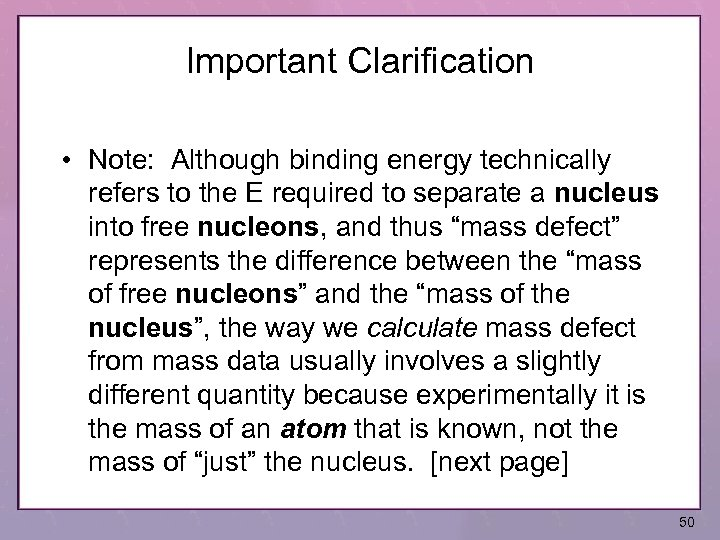Important Clarification • Note: Although binding energy technically refers to the E required to separate a nucleus into free nucleons, and thus “mass defect” represents the difference between the “mass of free nucleons” and the “mass of the nucleus”, the way we calculate mass defect from mass data usually involves a slightly different quantity because experimentally it is the mass of an atom that is known, not the mass of “just” the nucleus. [next page] 50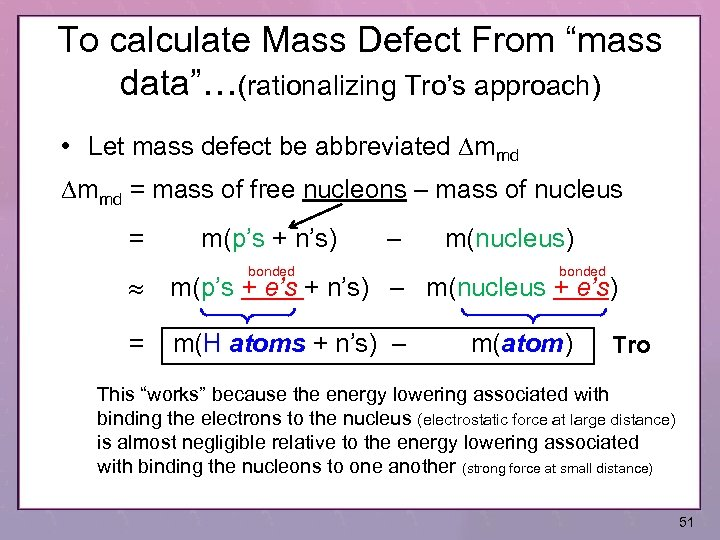To calculate Mass Defect From “mass data”…(rationalizing Tro’s approach) • Let mass defect be abbreviated Dmmd = mass of free nucleons – mass of nucleus = m(p’s + n’s) – m(nucleus) bonded m(p’s + e’s + n’s) – m(nucleus + e’s) = m(H atoms + n’s) – bonded m(atom) Tro This “works” because the energy lowering associated with binding the electrons to the nucleus (electrostatic force at large distance) is almost negligible relative to the energy lowering associated with binding the nucleons to one another (strong force at small distance) 51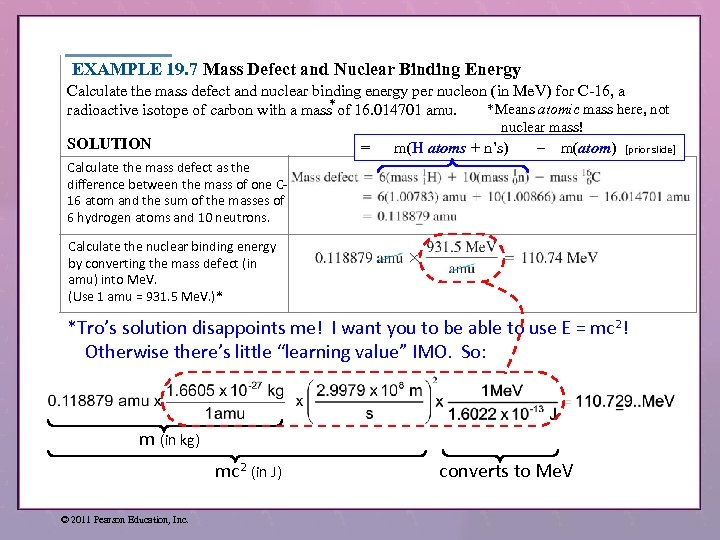EXAMPLE 19. 7 Mass Defect and Nuclear Binding Energy Calculate the mass defect and nuclear binding energy per nucleon (in Me. V) for C-16, a * *Means atomic mass here, not radioactive isotope of carbon with a mass of 16. 014701 amu. nuclear mass! SOLUTION = m(H atoms + n’s) – m(atom) [prior slide] Calculate the mass defect as the difference between the mass of one C 16 atom and the sum of the masses of 6 hydrogen atoms and 10 neutrons. Calculate the nuclear binding energy by converting the mass defect (in amu) into Me. V. (Use 1 amu = 931. 5 Me. V. )* *Tro’s solution disappoints me! I want you to be able to use E = mc 2! Otherwise there’s little “learning value” IMO. So: m (in kg) mc 2 (in J) © 2011 Pearson Education, Inc. converts to Me. V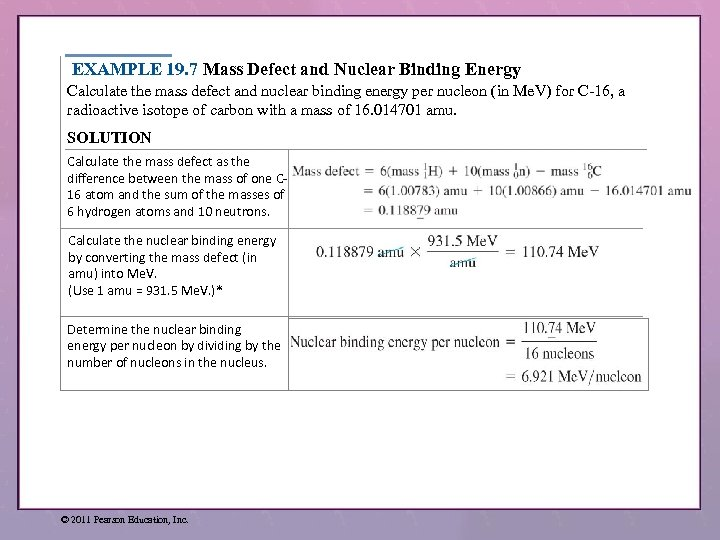EXAMPLE 19. 7 Mass Defect and Nuclear Binding Energy Calculate the mass defect and nuclear binding energy per nucleon (in Me. V) for C-16, a radioactive isotope of carbon with a mass of 16. 014701 amu. SOLUTION Calculate the mass defect as the difference between the mass of one C 16 atom and the sum of the masses of 6 hydrogen atoms and 10 neutrons. Calculate the nuclear binding energy by converting the mass defect (in amu) into Me. V. (Use 1 amu = 931. 5 Me. V. )* Determine the nuclear binding energy per nucleon by dividing by the number of nucleons in the nucleus. © 2011 Pearson Education, Inc.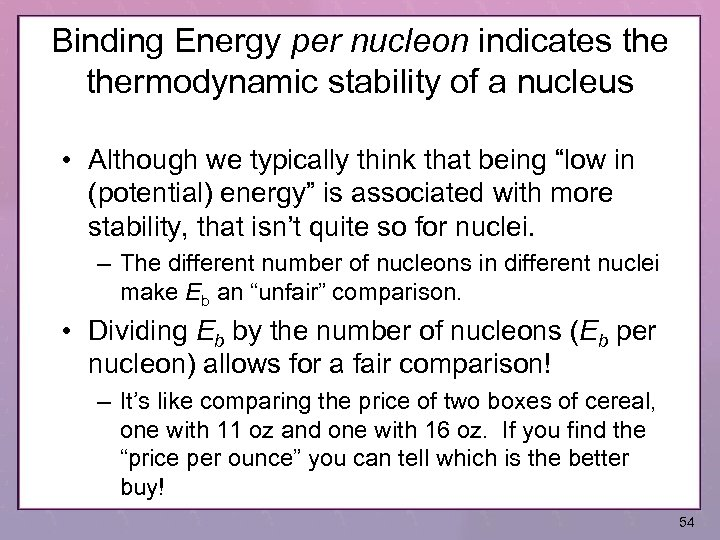Binding Energy per nucleon indicates thermodynamic stability of a nucleus • Although we typically think that being “low in (potential) energy” is associated with more stability, that isn’t quite so for nuclei. – The different number of nucleons in different nuclei make Eb an “unfair” comparison. • Dividing Eb by the number of nucleons (Eb per nucleon) allows for a fair comparison! – It’s like comparing the price of two boxes of cereal, one with 11 oz and one with 16 oz. If you find the “price per ounce” you can tell which is the better buy! 54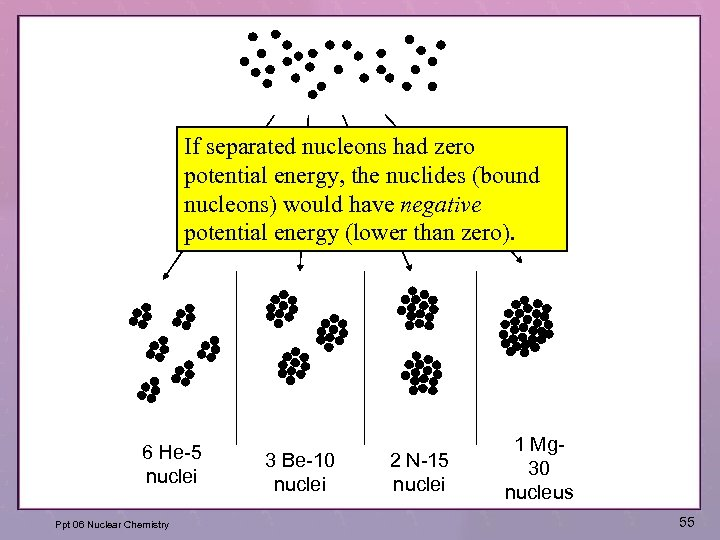If separated nucleons had zero potential energy, the nuclides (bound nucleons) would have negative potential energy (lower than zero). 6 He-5 nuclei Ppt 06 Nuclear Chemistry 3 Be-10 nuclei 2 N-15 nuclei 1 Mg 30 nucleus 55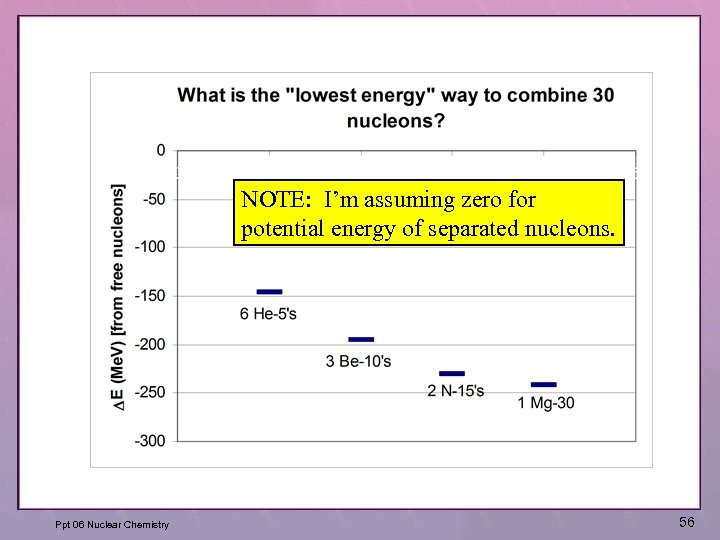NOTE: I’m assuming zero for potential energy of separated nucleons. Ppt 06 Nuclear Chemistry 56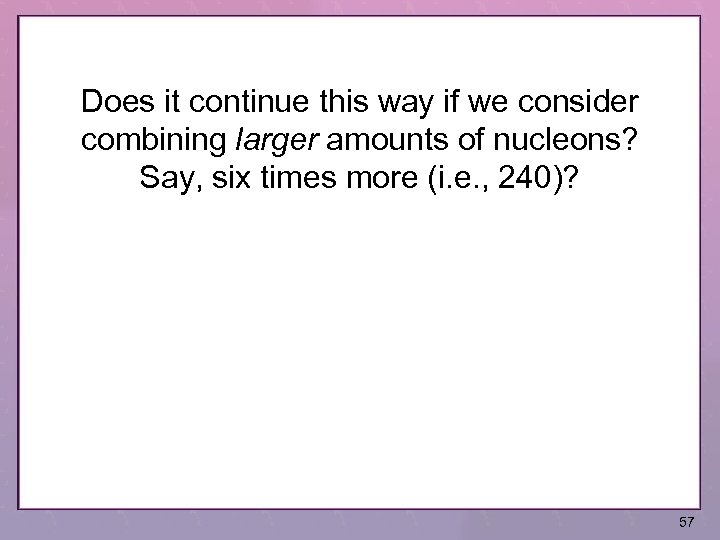Does it continue this way if we consider combining larger amounts of nucleons? Say, six times more (i. e. , 240)? 57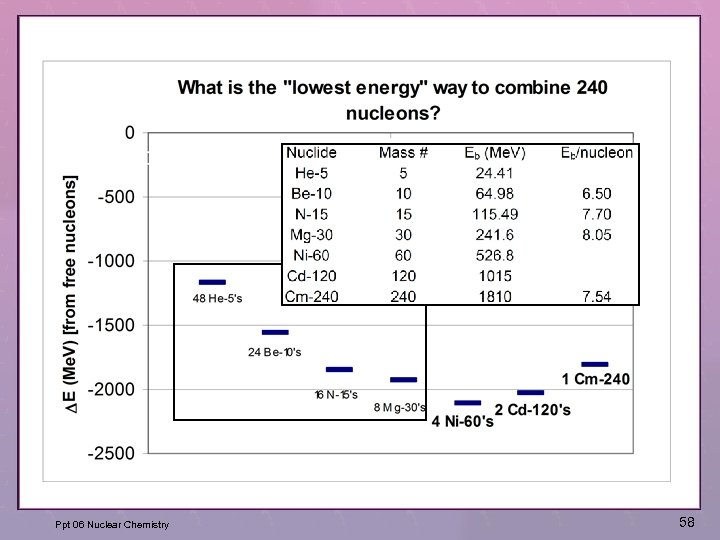Ppt 06 Nuclear Chemistry 58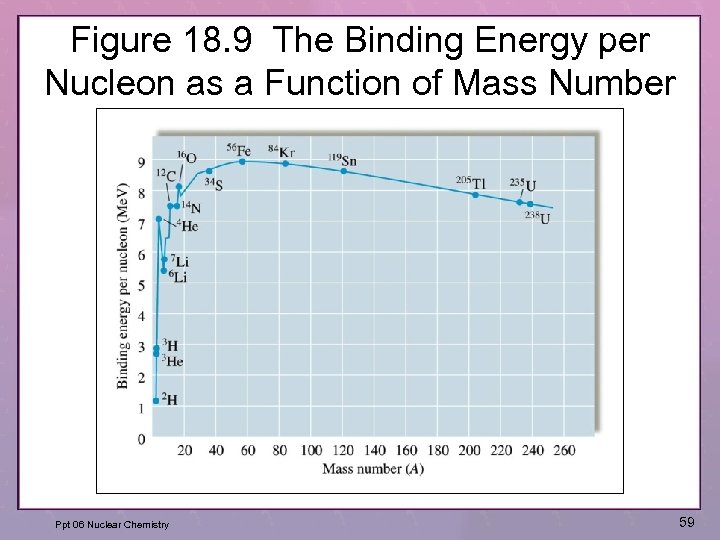Figure 18. 9 The Binding Energy per Nucleon as a Function of Mass Number Ppt 06 Nuclear Chemistry 59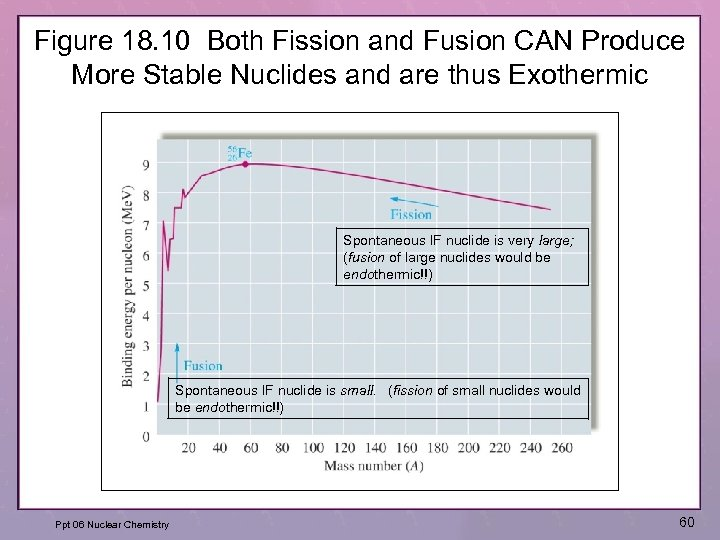Figure 18. 10 Both Fission and Fusion CAN Produce More Stable Nuclides and are thus Exothermic Spontaneous IF nuclide is very large; (fusion of large nuclides would be endothermic!!) Spontaneous IF nuclide is small. (fission of small nuclides would be endothermic!!) Ppt 06 Nuclear Chemistry 60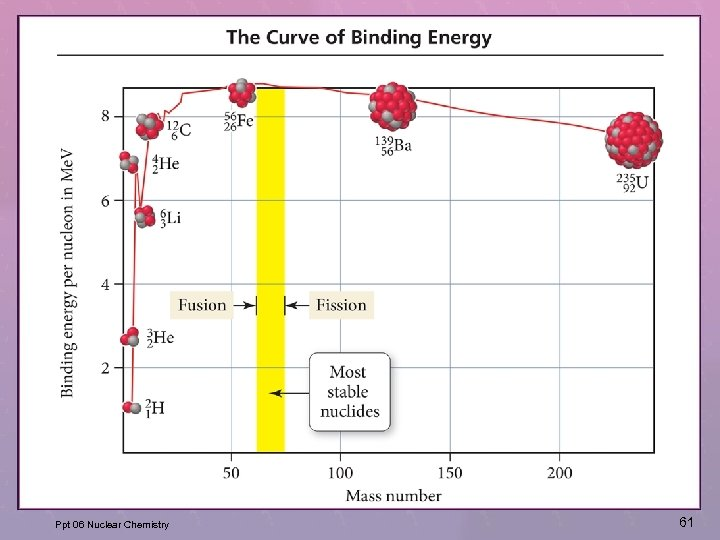Ppt 06 Nuclear Chemistry 61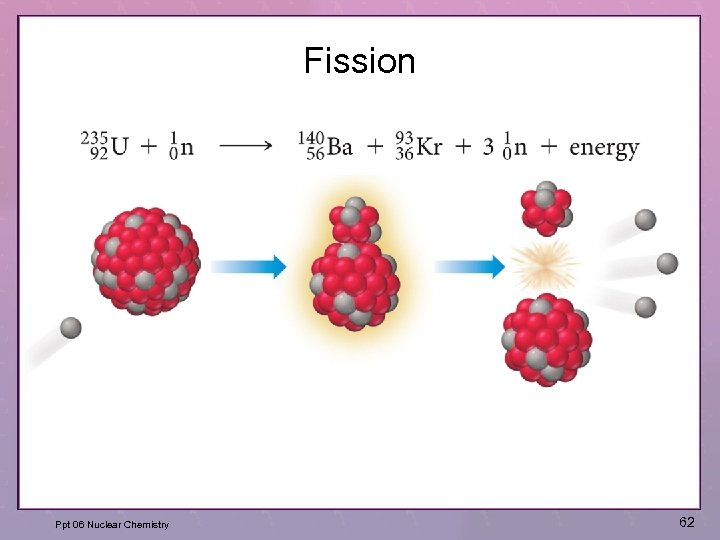Fission Ppt 06 Nuclear Chemistry 62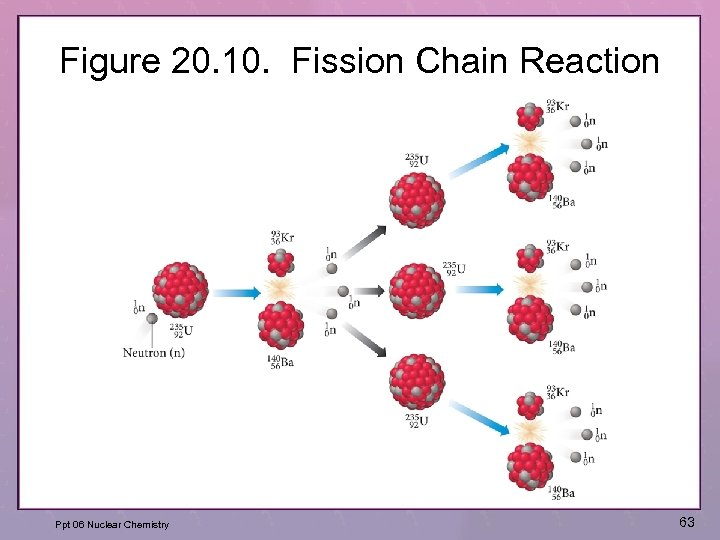Figure 20. 10. Fission Chain Reaction Ppt 06 Nuclear Chemistry 63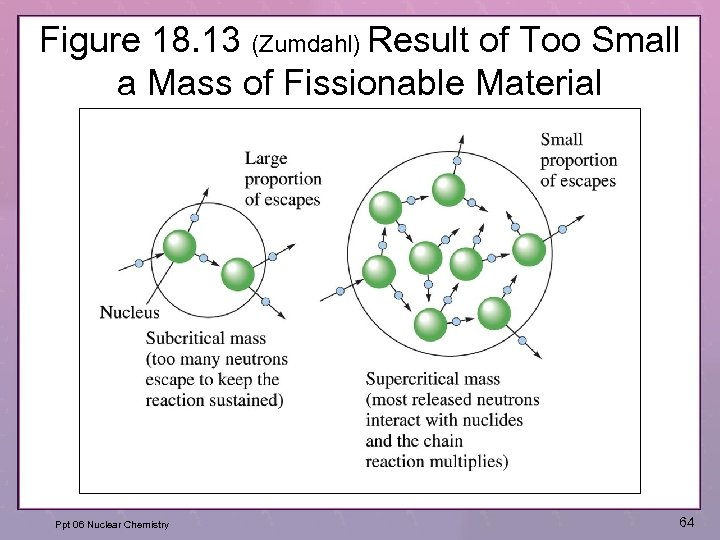Figure 18. 13 (Zumdahl) Result of Too Small a Mass of Fissionable Material Ppt 06 Nuclear Chemistry 64